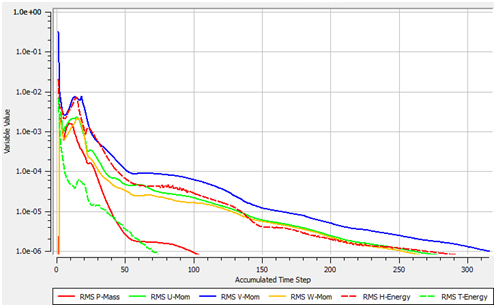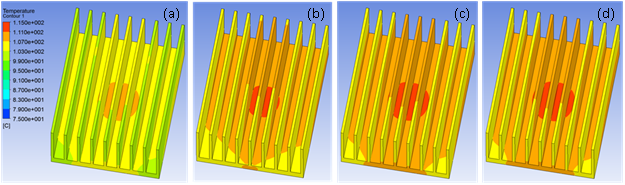# Has My CFD Analysis Converged? - Part IAugust 22, 2014 By: Michael Kuron

Practical fluid flow problems, by nature, are highly nonlinear - only under restrictive conditions can the governing Navier-Stokes equations be solved analytically. A consequence of this trait is that CFD solutions must be calculated iteratively, and as such, the point where we deem the solution "converged" is defined by the judgment of the user. So the question becomes: how do I know if my solution is converged? I typically use, at a minimum, the following three criteria to assess the convergence of a steady state CFD analysis: the residual level, the solution imbalances, and monitor points tracking quantities of interest as the analysis progresses. In Part I of this post, we'll discuss the use of residual values in judging convergence.

The residual is the most fundamental measure of convergence as it directly evaluates the error in the solution of a particular variable. The residual measures the local imbalance of a conserved variable in each control volume, so each control volume will have a residual value for each equation being solved. The lower the residual value is, the more numerically accurate the solution. Each code will have its own procedure for normalizing the solution residuals, so check your code’s documentation for guidance on an appropriate criteria when judging convergence.

In ANSYS CFX, RMS residual levels of 1E-4 are considered to be loosely converged, levels of 1E-5 are considered to be well converged, and levels of 1E-6 are considered to be tightly converged. For complicated problems, however, it's not always possible to achieve residual levels as low as 1E-6 or even 1E-5.So, let's take a look at the residual behavior in an example of a heat sink that sits atop a heat generating component being cooled by natural convection. The residual monitors in Figure 1 demonstrate monotonic convergence, indicating a well posed problem and a tightly converged solution. So how does the solution change as the residuals decrease? Figure 2 shows the temperature field in the heat sink at different residual levels.With the RMS residuals at 1E-4, the qualitative behavior of the heat sink can clearly be seen, but the peak temperature is underpredicted by as much as 5°C in this example. As the RMS residuals decrease to 5E-5, the temperature distribution begins to resemble the more tightly converged solutions and the peak temperature is predicted to within 1°C. Finally, as the solution further converges, the difference in the temperature distribution between residual levels of 1E-5 and 1E-6 is almost negligible.

I look forward to hearing your comments on the use of residuals to monitor solution quality. Stay Tuned for Part II, where I'll discuss the role of solution imbalances and monitor points in judging convergence.